# Rounding to the Nearest 10 and 100 for Grade 2 Students

## Understanding place value

Start by reviewing place value concepts with the students, focusing on hundreds and thousands. Explain that numbers are made up of digits, and each digit has a specific place value.

Place value is a fundamental concept in mathematics that helps us understand the numerical value of digits in a number based on their position or place within the number. Each digit in a number has a specific place value that determines its significance.

The place value system is based on powers of 10. In a base-10 system (which is the most common system used in everyday mathematics), the digits 0 to 9 are used, and the value of each digit depends on its position relative to the decimal point.

For example: 345

In this number, each digit has a specific place value:

The digit 3 is in the hundreds place (100).

The digit 4 is in the tens place (10).

The digit 5 is in the ones place (1).

To determine the value of each digit, we multiply the digit by its corresponding place value. Then, we sum up these values to find the overall value of the number:

Value = (3 × 100) + (4 × 10) + (5 × 1)

= 300 + 40 + 5

= 345

Now that your kids have grasped the concept of place value, it’s time to learn how to round numbers.

## Rounding to the Nearest 10

Identify the digit in the ones place of the number you want to round.

In rounding to the nearest 10, if the digit in the ones place is 0, 1, 2, 3, or 4, the number remains the same when rounded to the nearest 10. If it is 5, 6, 7, 8, or 9, it will be rounded up to the next multiple of 10.

Replace all the digits to the right of the ones place with zeros.

For example:

Let's say we want to round the number 36 to the nearest 10.

The digit in the ones place is 6, which is greater than 5, so we round up.

Replacing the digit in the ones place with a zero, we get 40.

So, 36 rounded to the nearest 10 is 40.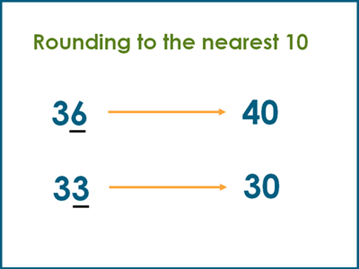## Rounding to the nearest 100

To help your kids, explain that when rounding to the nearest 100, we look at the tens digit. If the tens digit is 5 or greater, we round up; if it's less than 5, we round down.

Provide examples of three-digit numbers (e.g., 156, 247, 389) and explain that we can round them to the nearest 100.

Again, if the tens digit is 5 or greater, we round up; if it's less than 5, we round down.

Demonstrate the process using the above examples:

For 156: The tens digit is 5, so we round up to the nearest 100, which becomes 200.

For 247: The tens digit is 4, so we round down to the nearest 100, which becomes 200.

For 389: The tens digit is 9, so we round up to the nearest 100, which becomes 400.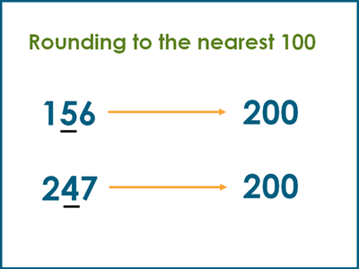## Reinforcement of learning to round to the nearest 10 and 100

In our grade 2 math section, we have several worksheets students can use to practice rounding to the nearest 10 and to the nearest 100.

## Rounding 2-digit numbers to the nearest 10

Students are presented with numbers between 10 and 99 to round to the nearest 10 in these worksheets.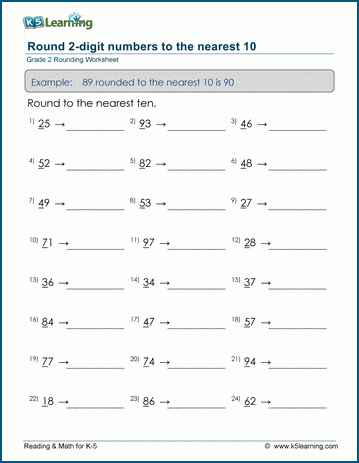## Rounding 3-digit numbers to the nearest 10

Students are presented with numbers between 100 and 999 to round to the nearest 10 in these worksheets.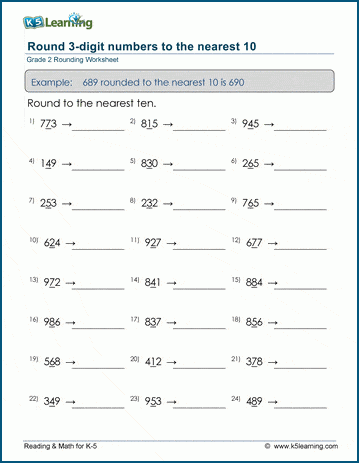## Rounding 3-digit numbers to the nearest 100

Next, students practice rounding to the nearest 100. They are presented with numbers between 100 and 1000 to practice.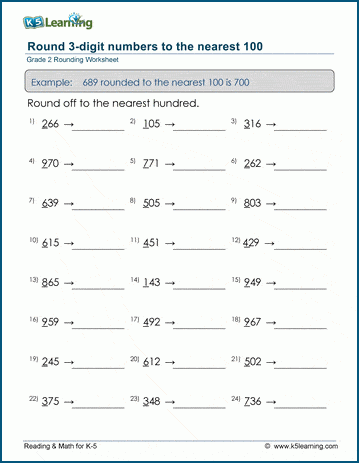## Round 3-digit numbers to the nearest 10 or 100

The final set of worksheets as students to round numbers to the nearest 10 or 100.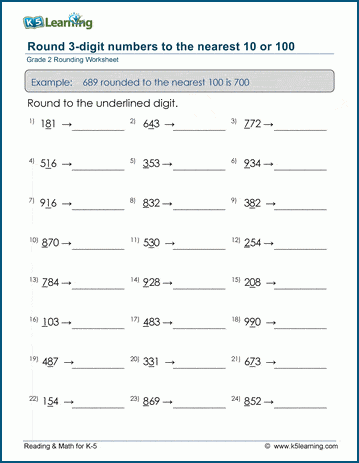Become a Member

This content is available to members only.